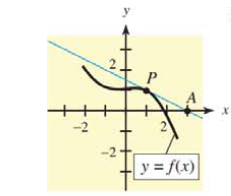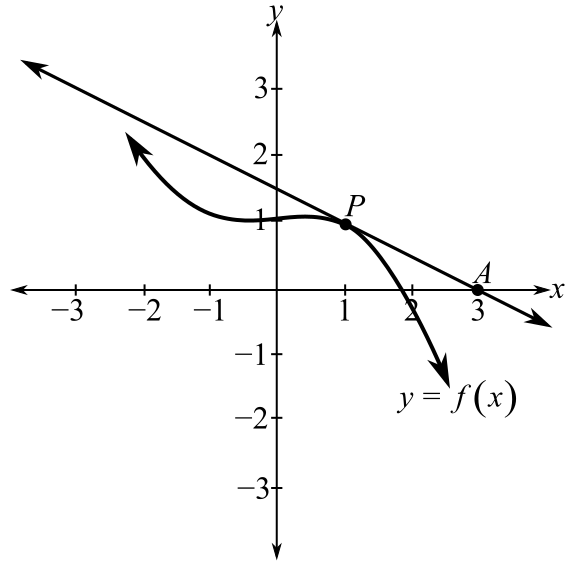Chapter 9.3, Problem 11E### Mathematical Applications for the ...

11th Edition
Ronald J. Harshbarger + 1 other
ISBN: 9781305108042

#### Solutions

Chapter
Section### Mathematical Applications for the ...

11th Edition
Ronald J. Harshbarger + 1 other
ISBN: 9781305108042
Textbook Problem

# In Problems 11-14, the tangent line to the graph of f(x) at x = 1 is shown. On the tangent line, P is the point of tangency and A is another point on the line.(a) Find the coordinates of the points P and A.(b) Use the coordinates of P and A to find the slope of the tangent line.(c) Find f'(l).(d) Find the instantaneous rate of change of f ( x ) at P.(a)

To determine

The coordinates of the points P and A if P is the point of tangency and A is a point on the tangent. The tangent line is drawn at x=1.Explanation

Given Information:

If P is the point of tangency and A is a point on the tangent.

The tangent line is drawn at x=1.

Explanation:

Consider the provided statement,

If P is the point of tangency and A is a point on the tangent. The tangent line is drawn at x=1.

Draw a perpendicular from point P parallel to x-axis. As seen from the graph, y=1

(b)

To determine

To calculate: The slope of the tangent line from points P and A as given in graph.(c)

To determine

To calculate: The value of f(1) if P is the point of tangency and A is a point on the tangent. The tangent line is drawn at x=1.(d)

To determine

To calculate: The instantaneous rate of change of y=f(x) at P if P is the point of tangency and A is a point on the tangent. The tangent line is drawn at x=1.### Still sussing out bartleby?

Check out a sample textbook solution.

See a sample solution

#### The Solution to Your Study Problems

Bartleby provides explanations to thousands of textbook problems written by our experts, many with advanced degrees!

Get Started

#### Find 26% of 360.

Elementary Technical Mathematics

#### Convert to higher terms as indicated. 11.

Contemporary Mathematics for Business & Consumers

#### True or False: If f′(c) = 0 and f″(c) < 0, then c is a local minimum.

Study Guide for Stewart's Single Variable Calculus: Early Transcendentals, 8th